# Important JEE type questions on linear momentum and system of particles

In this page we have Important JEE type questions on linear momentum and system of particles . Hope you like them and do not forget to like , social share and comment at the end of the page.
A mass of man m standing on a block of mass M. The system is at rest. The man moves relative in x direction to the block with velocity v' and then stops.
Question 1 Find the velocity of Centre of mass
(a) v'
(b) 0
(c)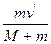(d) None of the above
Question 2   Find the displacement of block relative to ground if the displacement of the man with respect to block is xi
(a)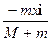(b)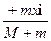(c) xi
(d) none of the above

Question 3 Find the horizontal component of the force with when acted on the block M  during the motion
(a)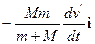(b)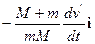(c)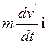(d) none of the above
A missile of mass M moving with velocity v= 200m/sec explodes in mid air breaking into two parts of mass M/4, 3M/4. If the smaller piece flies off at and angle of 60° with respect to the original direction with speed 400m/s.
Question 4 Find the velocity of the second piece
a) 100 m/s
b) 250 m/s
c) 300m/s
d) 231 m/s

Question 5 Q is defined as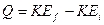What is the value of Q in this case?
(a)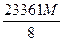(b)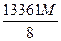(c)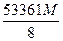(d) none of the above
Multiple Choice Questions

Question 6 Three bodies form an isolated system. There are m1 =m, m2 = 2m and m3 = 3m. They have different direction, but all have the same initial speed v0. One or more elastic collision between the pair of the bodies where otherwise do not interact. Find the maximum possible final speed of each of the three bodies.
(a) 3 v0, 2 v0, v0
(b) v0, 2 v0, 3 v0
(c) 2.4 v0, 1.73 v0, 1.41 v0
(d) none of the above
Question 7. A flat car of weight W roll without resistance along a horizontal surface .Initially a car together with a 3 men of weight w each is moving to the right with v0
Two cases are considered
Case1- All the three move simultaneously with velocity u relative to floor of car toward left. And jump off at the left
Case2- All the men run with relative velocity u and jump in succession towards left
Which of the following is true
(a) increase in velocity of flat car will be independent of the initial v0 of the system in both cases
(b) final velocity of flat car will be more in case2
(c) increment in velocity in case1 is given by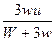(d) Increment in velocity in case2 after the second man jump is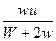Question 8. Let p1 and p2 be the momentum of two equal particles before elastic collision and p1 and p2 be the momentum after collision.
Mow we know p2 = 0
which of the following is true
(a)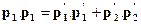(b)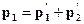(c)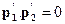(d) none of the above
Question 9. A body of mass M with small disc of mass m placed on its rest on a smooth horizontal plane.
The disc is set in motion in horizontal motion with velocity v.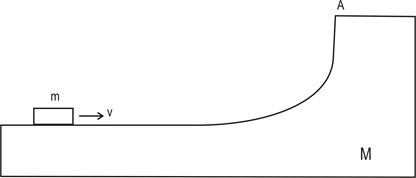which of the following is true
(a) the block M will starts moving towards due to constant force exerted by the disc m
(b) the height to which disc rise after breaking at point A from initial level is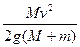(c) velocity of mass M when disc break from is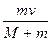(d) none of the above

Solutions